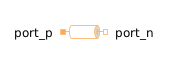Vector Gain - MapleSim Help

Vector Gain

Product of Vector and ScalarDescription The Vector Gain component multiplies a vector by a scalar.Equations $y=ku$Connections

 Name Description Modelica ID $y$ Real output vector y $k$ Real input signal k $u$ Real input vector uParameters

 Name Default Units Description Modelica ID $n$ $3$ dimension of input/output vectors nModelica Standard Library The component described in this topic is from the Modelica Standard Library. To view the original documentation, which includes author and copyright information, click here.